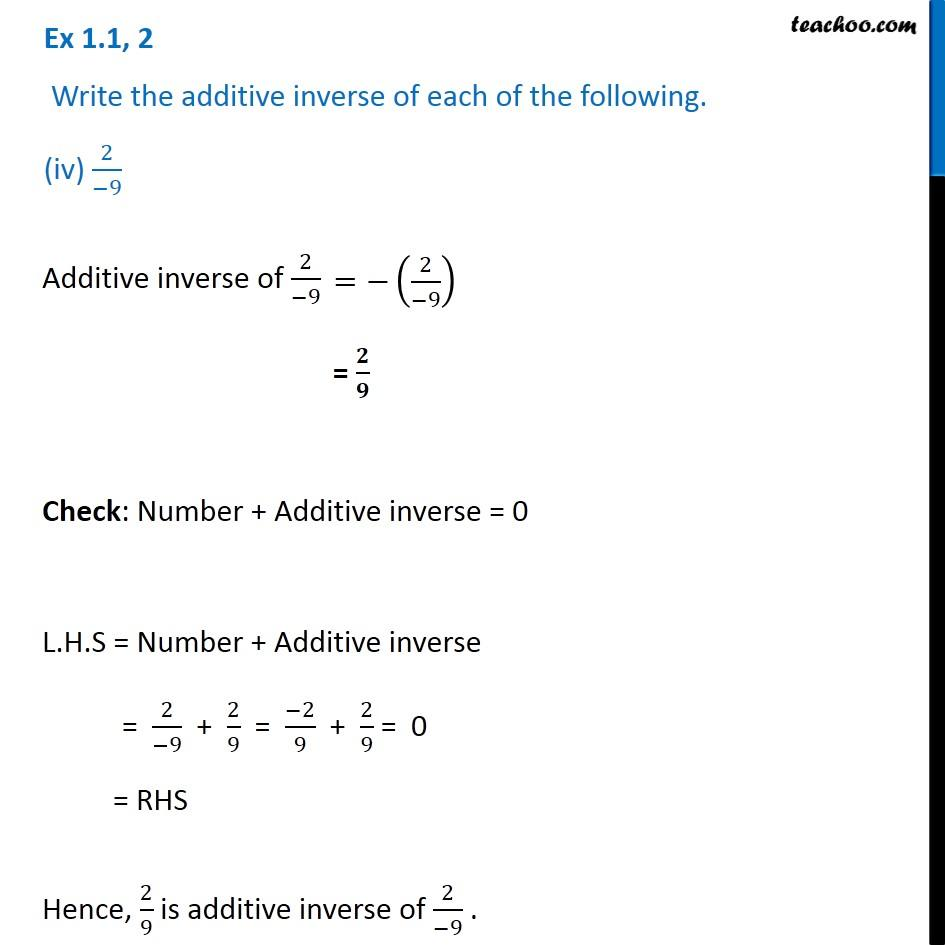Ex 1.1

Chapter 1 Class 8 Rational Numbers
Serial order wiseLearn in your speed, with individual attention - Teachoo Maths 1-on-1 Class

### Transcript

Ex 1.1, 2 Write the additive inverse of each of the following. (iv) 2/( 9) Additive inverse of 2/( 9) = / Check: Number + Additive inverse = 0 L.H.S = Number + Additive inverse = 2/( 9) + 2/9 = ( 2)/9 + 2/9 = 0 = RHS Hence, 2/9 is additive inverse of 2/( 9) .